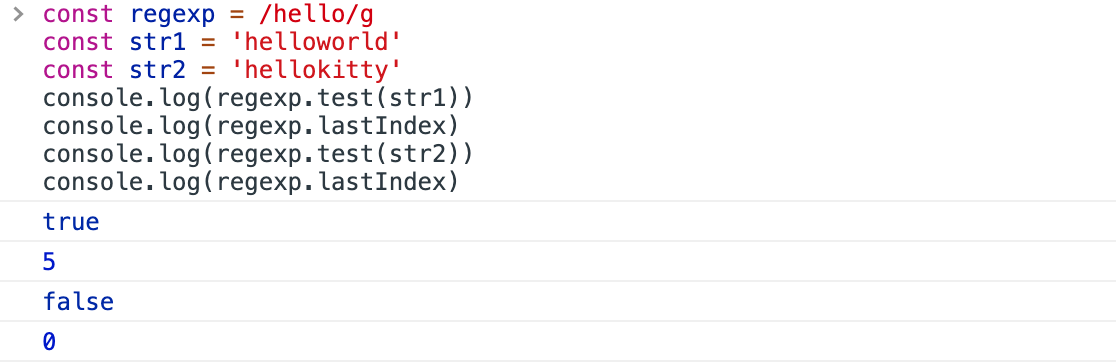## 问题重现

 ``````1 `````` ``````const regexp = /(?

 ``````1 2 `````` ``````const num = '1234567890' console.log(num.match(regexp)) ``````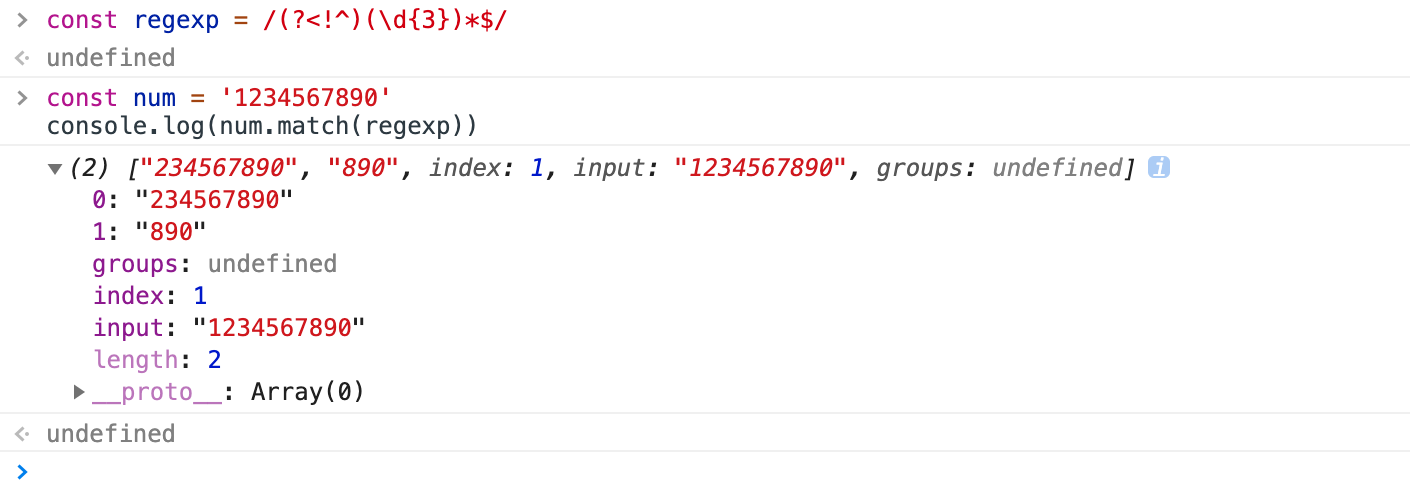``````1 2 3 `````` ``````const testStr = 'abc' const testReg = /(.)*/ console.log(testStr.match(testReg)) ``````

 ``````1 2 3 4 5 6 7 8 `````` ``````["abc", "c", index: 0, input: "abc", groups: undefined] 0: "abc" 1: "c" groups: undefined index: 0 input: "abc" length: 2 __proto__: Array(0) ``````

 ``````1 2 `````` ``````const testReg2 = /(.)(.)(.)/ console.log(testStr.match(testReg2)) ``````

 `````` 1 2 3 4 5 6 7 8 9 10 `````` ``````["abc", "a", "b", "c", index: 0, input: "abc", groups: undefined] 0: "abc" 1: "a" 2: "b" 3: "c" groups: undefined index: 0 input: "abc" length: 4 __proto__: Array(0) ``````

## 有什么影响

 ``````1 `````` ``````const url = 'http://www.mysite.com/path/sub/?xxx=xxx&yyy=yyy&zzz=zzz' ``````

 ``````1 `````` ``````const urlRegexp = /(https?):\/\/((?:\w+\.)+\w+)\/((?:\w+\/)*)\?((\w+=\w+&?)*)/ ``````

 ``````1 `````` ``````console.log(url.match(urlregexp)) ``````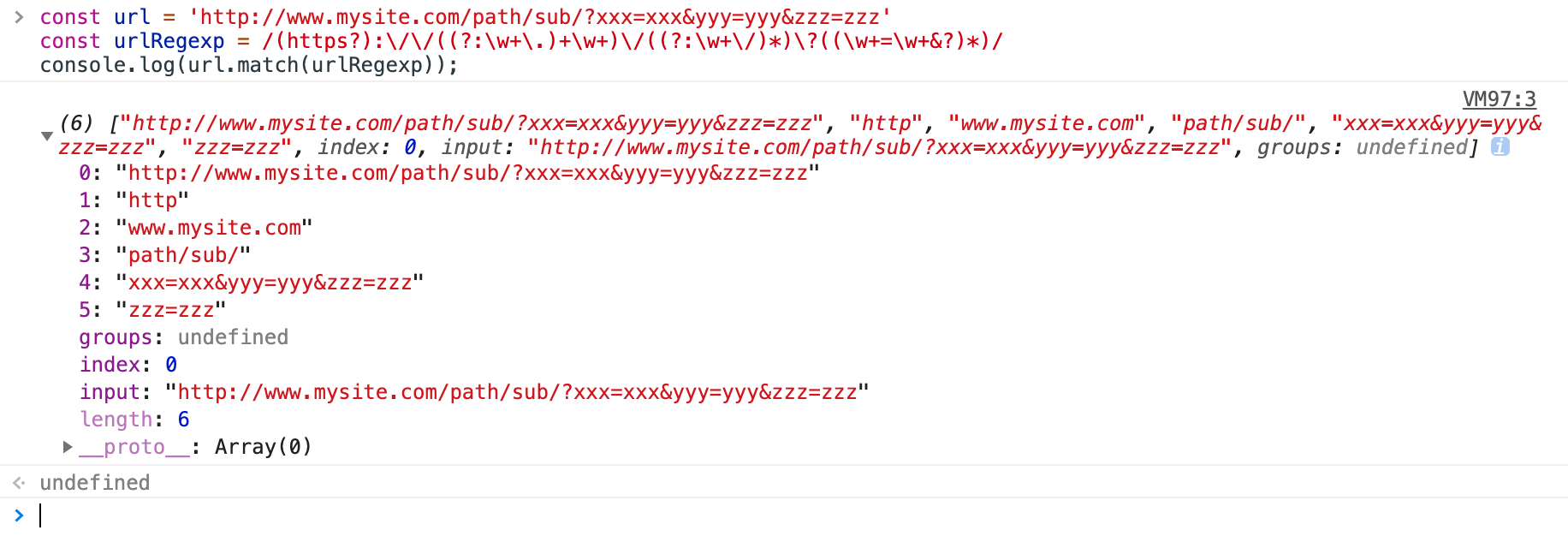## 这道题目的一个正则解答

• 不能是开头：(?<!^)
• 三个数字：(\d{3})
• 后面要正好是3的整数倍个数字：(?=(\d{3})*\$)

 ``````1 2 3 `````` ``````const newRegexp = /(?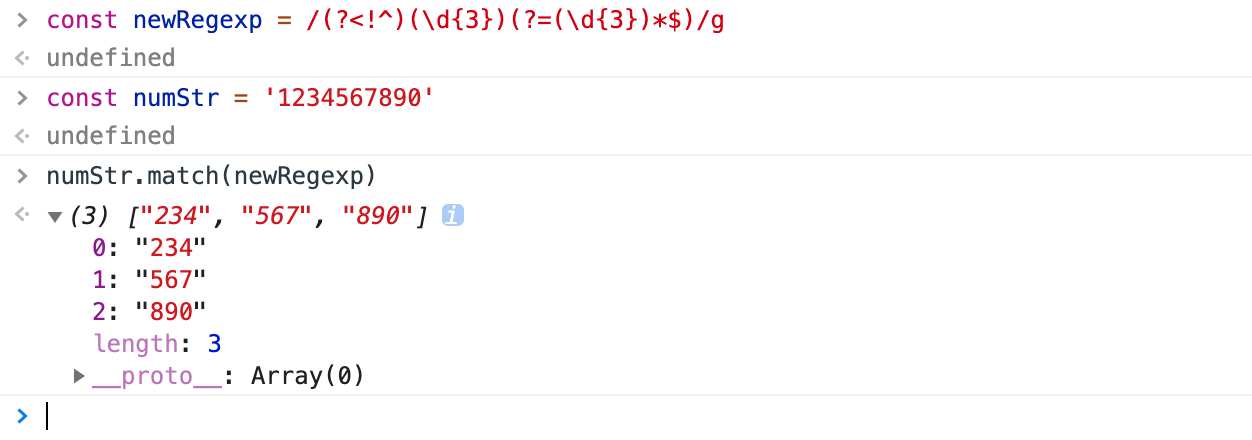``````1 2 3 4 `````` ``````function addComma(num) { const regexp=/(?

## 正则中的另一个问题

### 了解正则表达式的 lastIndex 属性

 `````` 1 2 3 4 5 6 7 8 9 10 11 12 `````` ``````const regexp = /hello/g const str = 'helloworld' console.log(regexp.test(str)) console.log(regexp.test(str)) console.log(regexp.test(str)) console.log(regexp.test(str)) console.log(regexp.test(str)) console.log(regexp.test(str)) console.log(regexp.test(str)) console.log(regexp.test(str)) console.log(regexp.test(str)) console.log(regexp.test(str)) ``````

 `````` 1 2 3 4 5 6 7 8 9 10 `````` ``````const regexp = /hello/g const str = 'helloworld' console.log(regexp.test(str)) console.log(regexp.lastIndex) console.log(regexp.test(str)) console.log(regexp.lastIndex) console.log(regexp.test(str)) console.log(regexp.lastIndex) console.log(regexp.test(str)) console.log(regexp.lastIndex) ``````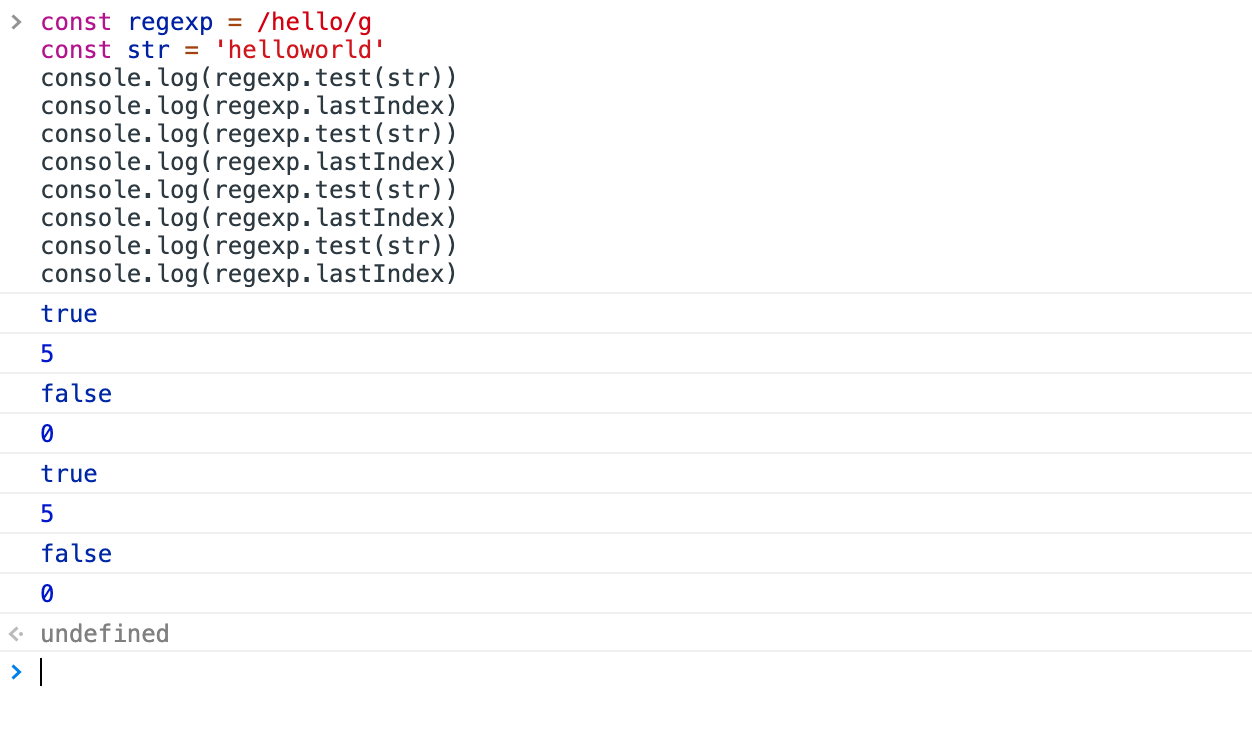有一点必须要了解，那就是这个属性是属于正则表达式对象的，如果你每次都使用不同的正则表达式对象，就不存在这个问题，这可以用一个函数来演示：

 ``````1 2 3 4 5 6 7 `````` ``````(function regexpTest(){ const str = 'helloworld' for (let i=0;i<10;i++){ const regexp = /hello/g console.log(regexp.test(str)) } })() ``````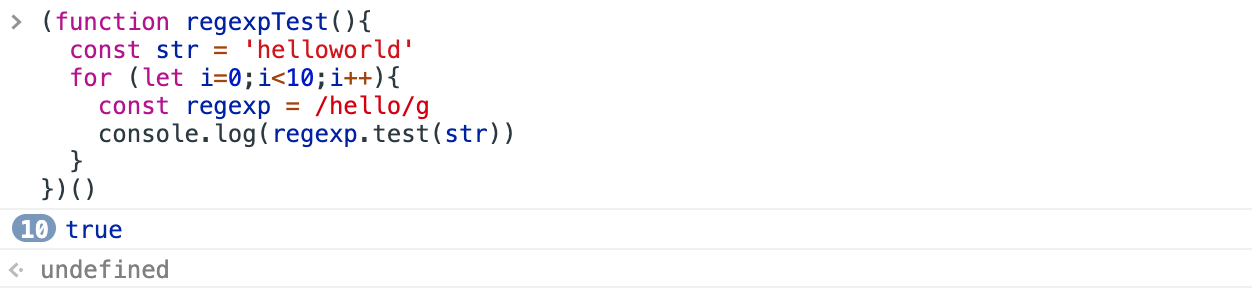### 问题演示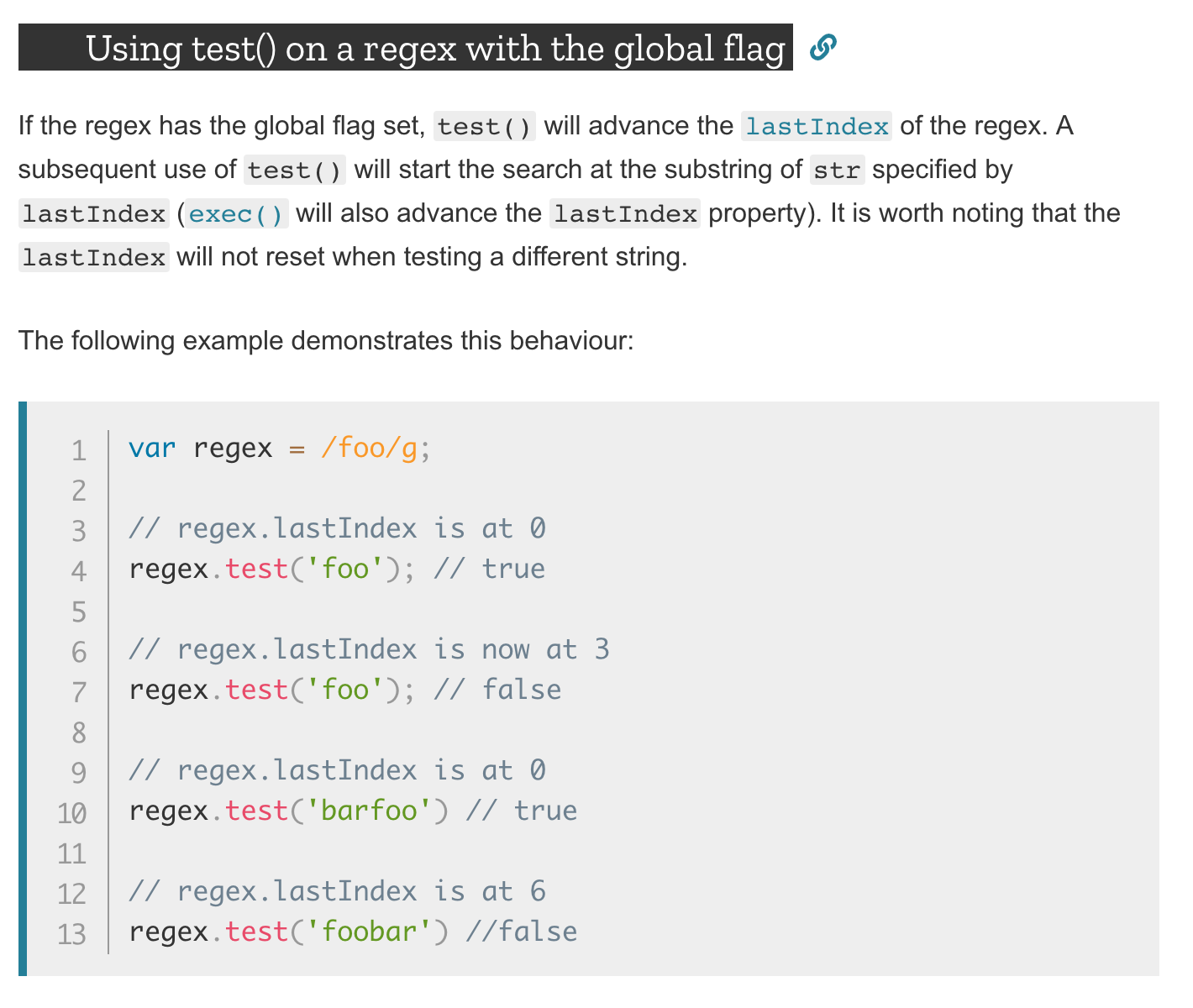``````1 2 3 4 5 6 7 `````` ``````const regexp = /hello/g const str1 = 'helloworld' const str2 = 'hellokitty' console.log(regexp.test(str1)) console.log(regexp.lastIndex) console.log(regexp.test(str2)) console.log(regexp.lastIndex) ``````